Courses

# NCERT Solutions - Thermodynamics, Class 11, Chemistry | EduRev Notes

## Chemistry Class 11

Created by: Mohit Rajpoot

## Class 11 : NCERT Solutions - Thermodynamics, Class 11, Chemistry | EduRev Notes

The document NCERT Solutions - Thermodynamics, Class 11, Chemistry | EduRev Notes is a part of the Class 11 Course Chemistry Class 11.
All you need of Class 11 at this link: Class 11

Ques 6.1: Choose the correct answer. A thermodynamic state function is a quantity
(i) used to determine heat changes
(ii) whose value is independent of path
(iii) used to determine pressure volume work
(iv) whose value depends on temperature only.
Ans:
A thermodynamic state function is a quantity whose value is independent of a path.
Functions like P, V, T etc. depend only on the state of a system and not on the path. Hence, alternative (ii) is correct.

Ques 6.2: For the process to occur under adiabatic conditions, the correct condition is:
(i) ΔT = 0
(ii) Δp = 0
(iii) q = 0
(iv) w = 0

Ans: A system is said to be under adiabatic conditions if there is no exchange of heat between the system and its surroundings. Hence, under adiabatic conditions, = 0.
Therefore, alternative (iii) is correct.

Ques 6.3: The enthalpies of all elements in their standard states are:
(i) unity
(ii) zero
(iii) < 0
(iv) different for each element
Ans:
The enthalpy of all elements in their standard state is zero.
Therefore, alternative (ii) is correct.

Ques 6.4:
ΔU° of combustion of methane is -X kJ mol–1. The value of ΔH° is
(i) = ΔU°
(ii) > ΔU°
(iii) < ΔU°
(iv) = 0
Ans 6.4:
Since ΔH° = ΔU° + ΔngRT and ΔU° = –X kJ mol–1,
ΔH° = (–X) + ΔngRT.
⇒ ΔH° < ΔU°
Therefore, alternative (iii) is correct.

Ques 6.5: The enthalpy of combustion of methane, graphite and dihydrogen at 298 K are, –890.3 kJ mol–1 –393.5 kJ mol–1, and –285.8 kJ mol–1 respectively. Enthalpy of formation of CH4(g) will be
(i) –74.8 kJ mol–1
(ii) –52.27 kJ mol–1
(iii) +74.8 kJ mol–1
(iv) +52.26 kJ mol–1.
Ans:
According to the question,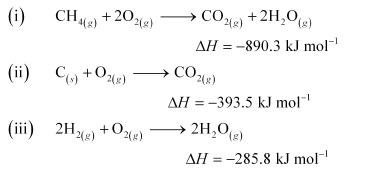Thus, the desired equation is the one that represents the formation of CH4 (g) i.e.,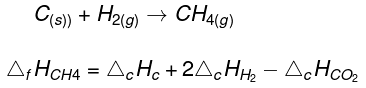= [-393.5 + 2(-285.8) - (-890.3)]kJ mol-1
=-74.8 kJ mol-1
∴ Enthalpy of formation of CH4(g) = -74.8 kJ mol-1
Hence, alternative (i) is correct.

Ques 6.6: A reaction, A + B → C + D + q is found to have a positive entropy change. The reaction will be
(i) possible at high temperature
(ii) possible only at low temperature
(iii) not possible at any temperature
(iv) possible at any temperature
For a reaction to be spontaneous, ΔG should be negative.
ΔG = ΔHTΔS
According to the question, for the given reaction,
ΔS = positive
ΔH = negative (since heat is evolved)
⇒ ΔG = negative
Therefore, the reaction is spontaneous at any temperature.
Hence, alternative (iv) is correct.

Ques 6.7: In a process, 701 J of heat is absorbed by a system and 394 J of work is done by the system. What is the change in internal energy for the process
Ans:
According to the first law of thermodynamics,
ΔU = q + W (i)
Where,
ΔU = change in internal energy for a process
q = heat
W = work
Given,
q = + 701 J (Since heat is absorbed)
W = –394 J (Since work is done by the system)
Substituting the values in expression (i), we get
ΔU = 701 J + (–394 J)
ΔU = 307 J
Hence, the change in internal energy for the given process is 307 J.

Ques 6.8: The reaction of cyanamide, NH2CN(s),with dioxygen was carried out in a bomb calorimeter, and Δwas found to be –742.7 kJ mol–1at 298 K. Calculate enthalpy change for the reaction at 298 K.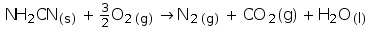Ans:
Enthalpy change for a reaction (ΔH) is given by the expression,
ΔH = ΔU + ΔngRT
Where,
ΔU = change in internal energy
Δng = change in number of moles
For the given reaction,
Δng = ∑ng (products) – ∑ng (reactants)
= (2 – 1.5) moles
Δng = 0.5 moles
And,
ΔU = –742.7 kJ mol–1
T = 298 K
R = 8.314 × 10–3 kJ mol–1 K–1
Substituting the values in the expression of ΔH:
ΔH = (–742.7 kJ mol–1) + (0.5 mol) (298 K) (8.314 × 10–3 kJ mol–1 K–1)
= –742.7 + 1.2
ΔH = –741.5 kJ mol–1

Ques 6.9: Calculate the number of kJ of heat necessary to raise the temperature of 60.0 g of aluminium from 35°C to 55°C. Molar heat capacity of Al is 24 J mol–1 K–1.
Ans:
From the expression of heat (q),
q = m. c. ΔT
Where,
c = molar heat capacity
m = mass of substance
ΔT = change in temperature
Substituting the values in the expression of q: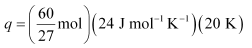q = 1066.7 J
q = 1.07 kJ

Ques 6.10: Calculate the enthalpy change on freezing of 1.0 mol of water at 10.0°C to ice at –10.0°C. Δfus= 6.03 kJ mol–1 at 0°C.
Cp[H2O(l)] = 75.3 J mol–1 K–1
Cp[H2O(s)] = 36.8 J mol–1 K–1
Ans:
Total enthalpy change involved in the transformation is the sum of the following changes:
(a) Energy change involved in the transformation of 1 mol of water at 10°C to 1 mol of water at 0°C.
(b) Energy change involved in the transformation of 1 mol of water at 0° to 1 mol of ice at 0°C.
(c) Energy change involved in the transformation of 1 mol of ice at 0°C to 1 mol of ice at –10°C.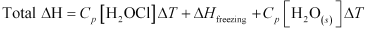= (75.3 J mol–1 K–1) (0 – 10)K + (–6.03 × 103 J mol–1) + (36.8 J mol–1 K–1) (–10 – 0)K
= –753 J mol–1 – 6030 J mol–1 – 368 J mol–1
= –7151 J mol–1
= –7.151 kJ mol–1
Hence, the enthalpy change involved in the transformation is –7.151 kJ mol–1.

Ques 6.11: Enthalpy of combustion of carbon to CO2 is –393.5 kJ mol–1. Calculate the heat released upon formation of 35.2 g of CO2 from carbon and dioxygen gas.
Ans:
Formation of CO2 from carbon and dioxygen gas can be represented as: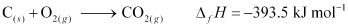(1 mole = 44 g)
Heat released on formation of 44 g CO2 = –393.5 kJ mol–1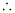Heat released on formation of 35.2 g CO2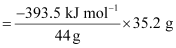= –314.8 kJ mol–1

Ques 6.12: Enthalpies of formation of CO(g), CO2(g), N2O(gand N2O4(gare –110 kJ mol–1, – 393 kJ mol–1, 81 kJ mol–1and 9.7 kJ mol–1 respectively. Find the value of Δrfor the reaction:

N2O4(g) + 3CO(g)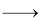N2O(g) + 3CO2(g)
Ans
ΔrH for a reaction is defined as the difference between ΔfH value of products and ΔfH value of reactants.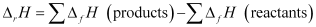For the given reaction,

N2O4(g) + 3CO(g)N2O(g) + 3CO2(g)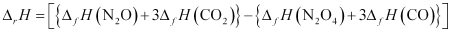Substituting the values of ΔfH for N2O, CO2, N2O4, and CO from the question, we get: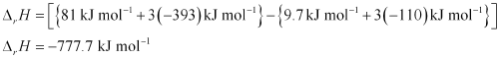Hence, the value of Δrfor the reaction is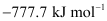.

Ques 6.13:
Given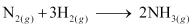; ΔrHθ = –92.4 kJ mol–1
What is the standard enthalpy of formation of NH3 gas?
Ans:
Standard enthalpy of formation of a compound is the change in enthalpy that takes place during the formation of 1 mole of a substance in its standard form from its constituent elements in their standard state.
Re-writing the given equation for 1 mole of NH3(g),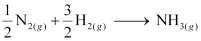Standard enthalpy of formation of NH3(g)
= ½ ΔrHθ
= ½ (–92.4 kJ mol–1)
= –46.2 kJ mol–1

Ques 6.14:
Calculate the standard enthalpy of formation of CH3OH(l) from the following data:
CH3OH(l) +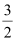O2(g)CO2(g) + 2H2O(l) ; ΔrHθ = –726 kJ mol–1
C(g) + O2(g)CO2(g) ; ΔcHθ = –393 kJ mol–1
H2(g) +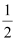O2(g)H2O(l) ; ΔfHθ = –286 kJ mol–1.

Ans
The reaction that takes place during the formation of CH3OH(l) can be written as:
C(s) + 2H2O(g) +O2(g)CH3OH(l) (1)

The reaction (1) can be obtained from the given reactions by following the algebraic calculations as:
Equation (ii) + 2 × equation (iii) – equation (i)
ΔfHθ [CH3OH(l)] = ΔcHθ + 2ΔfHθ [H2O(l)] – ΔrHθ
= (–393 kJ mol–1) + 2(–286 kJ mol–1) – (–726 kJ mol–1)
= (–393 – 572 + 726) kJ mol–1ΔfHθ [CH3OH(l)] = –239 kJ mol–1

Ques 6.15: Calculate the enthalpy change for the process
CCl4(g) → C(g) + 4Cl(g
and calculate bond enthalpy of C–Cl in CCl4(g).
ΔvapHθ (CCl4) = 30.5 kJ mol–1.
ΔfHθ (CCl4) = –135.5 kJ mol–1.
ΔaHθ (C) = 715.0 kJ mol–1, where ΔaHθ is enthalpy of atomisation
ΔaHθ (Cl2) = 242 kJ mol–1
Ans:
The chemical equations implying to the given values of enthalpies are: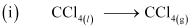ΔvapHθ = 30.5 kJ mol–1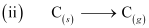ΔaHθ = 715.0 kJ mol–1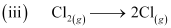ΔaHθ = 242 kJ mol–1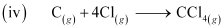ΔfH = –135.5 kJ mol–1
Enthalpy change for the given process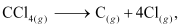can be calculated using the following algebraic calculations as:
Equation (ii) + 2 × Equation (iii) – Equation (i) – Equation (iv)
ΔH = ΔaHθ(C) + 2ΔaHθ (Cl2) – ΔvapHθ – ΔfH
= (715.0 kJ mol–1) + 2(242 kJ mol–1) – (30.5 kJ mol–1) – (–135.5 kJ mol–1)ΔH = 1304 kJ mol–1
Bond enthalpy of C–Cl bond in CCl4 (g)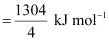= 326 kJ mol–1

Ques 6.16: For an isolated system, Δ= 0, what will be ΔS?
Ans:
ΔS will be positive i.e., greater than zero
Since ΔU = 0, ΔS will be positive and the reaction will be spontaneous.

Ques 6.17: For the reaction at 298 K,
2A + B → C
Δ= 400 kJ mol–1 and Δ= 0.2 kJ K–1 mol–1
At what temperature will the reaction become spontaneous considering Δand Δto be constant over the temperature range?

Ans:

From the expression,
ΔG = ΔHTΔS
Assuming the reaction at equilibrium, ΔT for the reaction would be: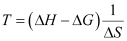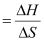(ΔG = 0 at equilibrium)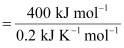T = 2000 K
For the reaction to be spontaneous, ΔG must be negative. Hence, for the given reaction to be spontaneous, T should be greater than 2000 K.

Ques 6.18:
For the reaction,
2Cl(g) → Cl2(g), what are the signs of Δand Δ?
Ans:
ΔH and Δare negative
The given reaction represents the formation of chlorine molecule from chlorine atoms. Here, bond formation is taking place. Therefore, energy is being released. Hence, ΔH is negative.
Also, two moles of atoms have more randomness than one mole of a molecule. Since spontaneity is decreased, ΔS is negative for the given reaction.

Ques 6.19: For the reaction
2A(g) + B(g) → 2D(g)
ΔUθ = –10.5 kJ and ΔSθ= –44.1 JK–1.
Calculate ΔGθ for the reaction, and predict whether the reaction may occur spontaneously.
Ans:
For the given reaction,
2 A(g) + B(g) → 2D(g)
Δng = 2 – (3)
= –1 mole
Substituting the value of ΔUθ in the expression of ΔH:
ΔHθ = ΔUθ + ΔngRT
= (–10.5 kJ) – (–1) (8.314 × 10–3 kJ K–1 mol–1) (298 K)
= –10.5 kJ – 2.48 kJ
ΔHθ = –12.98 kJ
Substituting the values of ΔHθ and ΔSθ in the expression of ΔGθ:
ΔGθ = ΔHθTΔSθ
= –12.98 kJ – (298 K) (–44.1 J K–1)
= –12.98 kJ + 13.14 kJ
ΔGθ = + 0.16 kJ
Since ΔGθ for the reaction is positive, the reaction will not occur spontaneously.

Ques 6.20: The equilibrium constant for a reaction is 10. What will be the value of ΔGθ? R = 8.314 JK–1 mol–1, T = 300 K.
Ans:
From the expression,
ΔGθ = –2.303 RT logKeq
ΔGθ for the reaction,
= (2.303) (8.314 JK–1 mol–1) (300 K) log10
= –5744.14 Jmol–1
= –5.744 kJ mol–1

Ques 6.21: Comment on the thermodynamic stability of NO(g), given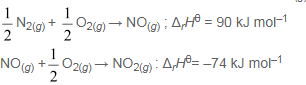Ans:
The positive value of ΔrH indicates that heat is absorbed during the formation of NO(g). This means that NO(g) has higher energy than the reactants (N2 and O2). Hence, NO(g) is unstable.
The negative value of ΔrH indicates that heat is evolved during the formation of NO2(g) from NO(g) and O2(g). The product, NO2(g) is stabilized with minimum energy.
Hence, unstable NO(g) changes to stable NO2(g).

Ques 6.22:Calculate the entropy change in surroundings when 1.00 mol of H2O(l) is formed under standard conditions. ΔfHθ = –286 kJ mol–1.
Ans:
It is given that 286 kJ mol–1 of heat is evolved in the formation of 1 mol of H2O(l). Thus, an equal amount of heat will be absorbed by the surroundings.
qsurr = +286 kJ mol–1

Entropy change (ΔSsurr) for the surroundings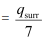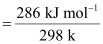ΔSsurr = 959.73 J mol–1 K–1

,

,

,

,

,

,

,

,

,

,

,

,

,

,

,

,

,

,

,

,

,

,

,

,

,

,

,

;